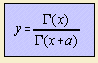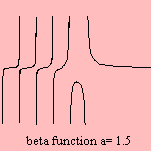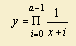# beta function

## gammaGiven the gamma function, a function of two variables can be derived: the beta function: Β(a,b) to be defined as: Γ(a) Γ(b) / Γ(a+b).
As the gamma function is defined as an integral, the beta function can also be written in an integral form 1).

The name of the curve is given by Binet (1839). But it has been studied before, by Euler (1771) and Legendre (1809). So that the curve is also called the Euler beta function or the first Euler function.

For positive integer values for the parameter a the beta function reduces to a polynomial (in x and y) 2).

The beta function comes into picture when calculating the total density by performing convolutions of classical state densities which have a power law form. An example is the situation of two subsystems composed of different sets of harmonic oscillators s1 and s2. Then the density is a function of Β(s1, s2).

notes

1)2)